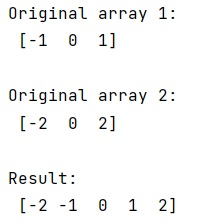# Concatenate Two Arrays and Extract Unique Values in Python

In this tutorial, we will learn how to concatenate two arrays and extract unique values in Python? By Pranit Sharma Last updated : May 13, 2023

Given two NumPy array arr1 and arr2, we have to concatenate them and extract the unique values.

Example:

```Input:
arr1: [-1, 0, 1]
arr2: [-2, 0, 2]

Output:
[2 , -1, 0, 1, 2]
```

## How to concatenate two arrays and extract unique values?

To concatenate two arrays and extract unique values, the easiest way is to use the numpy.union1d() method, it performs the union operation on one-dimensional arrays, and returns the unique, sorted array of values that are in either of the two input arrays.

Let us understand with the help of an example,

## Python program to concatenate two arrays and extract unique values

```# Import numpy
import numpy as np

# Creating numpy arrays
arr1 = np.array([-1, 0, 1])
arr2 = np.array([-2, 0, 2])

# Display original arrays
print("Original array 1:\n", arr1, "\n")
print("Original array 2:\n", arr2, "\n")

# Concatenating arrays and finding unique values
res = np.union1d(arr1, arr2)

# Display result
print("Result:\n", res, "\n")
```

### Output

```Original array 1:
[-1  0  1]

Original array 2:
[-2  0  2]

Result:
[-2 -1  0  1  2]
```

### Output (Screenshot)